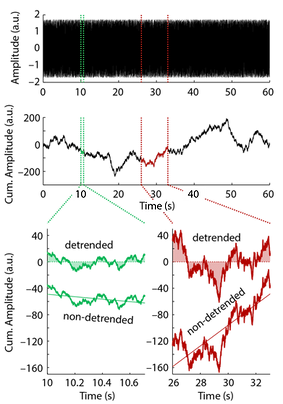top of page

## Scale-free systems and variability

Investigations of neural signals are often described in terms of an average that discards the observed variability. An average measure, however, might be a poor description of a neural signal, if the signal does not have a typical scale. These systems are referred to as scale-free (for a good review see Hardstone et al. 2012).

More and more research aims at characterizing scale-free signals. It has attracked attention particularly in the fields that study rhythmic (oscillations) and non-rhythmic neural activity. Previous investigations have revealed changes in non-rhythmic neural activity as a function of age (Voytek et al. 2015) and task (He et al. 2010), and log-range correlations in neural signals (Linkenkaer-Hansen et al. 2001).

One of the challenges researchers face is how to assess noise/variability or scale-free properties in neural signals. In the following, we describe the detrended fluctuation analysis, which quantifies the long-term autocorrelation or long-range correlation of non-stationary signals (Hardstone et al. 2012, Linkenkaer-Hansen et al. 2001). Please see also the tutorial on multi-scale entropy which is closely related to the detrended fluctuation analysis and also quantifies variablity in time series.

## Simulated time series with different 1/f structure

For the simulations discussed below, four sample time series with a duration of 60 s were generated. The four time series differ in their (1/f) noise structure: blue noise (1/f^-1), white noise (1/f^0), pink noise (1/f^1), brown noise (1/f^2). The time courses and corresponding spectra are shown on the right. Low-frequency power increases and high-frequency power decreases as the 1/f exponent increases.

Time series may also be derived from the neural power fluctuations over time calculated using time-frequency transformations of the signal (e.g., by means of wavelet analysis).## Detrended fluctuation analysis: The method

The detrended fluctuation analysis method is well descibed in empirical and review papers (Hardstone et al.). The method can be applied to any given time series (although the time series should not be too short) and involves the following steps. The mean is calculated across all time points of the time series and then subtracted from the signal at each time point.

This centers the signal around zero (the top panel of the figure on the left shows the mean-centered 'white noise' time series). The cumulative sum is calculated for the mean-centered time series (displayed in the middle panel of the figure). Sliding windows of different size are subsequently shifted across the cumulative sum signal with a 50% overlap. Window sizes are usually logarithmically spaced. Here, 20 windows were used and their size ranged from 0.1 s to 50 s (the time course of the cumulative sum for two example windows are displayed in the bottom panel). For each window shift, the signal is detrended using linear regression (i.e., slope and intercept are removed; bottom panel) which mean-centers the signal within a window.The root-mean-square (RMS) is then calculated for the detended signal (sometimes the standard deviation is used instead). The root-mean square is closely related to the sum over the area between the signal and zero (shaded area in the bottom panel of the figure on the left). The root-mean-square is averaged across all shifts of a particular window size. This results in a mean root-mean-square value for each window size, which, when displayed on logarithmic axes, shows a linear trend (shown in the figure on the right; the color dots relate to the time windows displayed in the figure on the left). Finally, a linear function is fit to the log-transformed root-mean square values as a function of the log-transformed window size. The estimated slope (here called alpha coefficient) of the fitted linear function reflects the degree long-range correlation in the orginal time series (the bar graph in the figure on the right shows the slope/alpha). We show below how slope/alpha depends on the type of 1/f structure in time series## Detrended fluctuation analysis: Results for different noise types

We used the detrended fluctuation analysis to calculate the alpha coefficient for the four different noise types described above. The alpha coefficient (i.e., the slope of the linear function fit to the RMS values; see figure on the left) increases parametrically with the 1/f exponent that was used to generate the time series. White noise (1/f^0) has an alpha coefficient of about 0.5. and pink noise (1/f^1) has an alpha coefficient of about 1. The alpha coefficients indicate that the different time series differ in their scaling properties. They quantify the long-term autocorrelation or long-range correlation of non-stationary signals (Hardstone et al. 2001, Linkenkaer-Hansen et al. 2012). Intuitively, long-range (auto-) correlations are larger with more energy in the low-frequency parts of a signal.

A matlab script that calculates the detrended fluctuation analysis can be downloaded here. Please only use under under GNU license. No warranty can be provided. Self-check for errors.

bottom of page